# AP Statistics : How to find sampling distribution of a sample proportion

## Example Questions

### Example Question #1 : Properties Of Single Sample Distributions

A researcher wants to determine whether there is a significant linear relationship between time spent meditating and time spent studying. What is the appropriate null hypothesis for this study?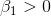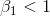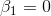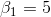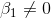Explanation:

This question is about a linear regression between time spent meditating and time spent studying. Therefore, the hypothesis is regarding Beta1, the slope of the line. We are testing a non-directional or bi-directional claim that the relationship is significant. Therefore, the null hypothesis is that the relationship is not significant, meaning the slope of the line is equal to zero.

Explanation:

Explanation:

### Example Question #4 : Properties Of Single Sample Distributions

The president of a country is trying to estimate the average income of his citizens. He randomly samples residents and collects information about their salaries. A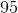percent confidence interval computed from this data for the mean income per citizen is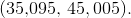Which of the following provides the best interpretation of this confidence interval?

There is apercent probability that the mean of another sample with the same size will fall between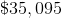and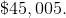percent of the citizens of the country have an income that is betweenandThere is apercent probability that the mean income per citizen in the school is betweenandIf he was to take another sample of the same size and compute apercent confidence interval, we would have apercent chance of getting the intervalThere is apercent probability that all the citizens of the country have an income  betweenandThere is apercent probability that the mean income per citizen in the school is betweenandA confidence interval is a statement about the mean of the population the sample is drawn from so there is apercent probability that apercent confidence interval contains the true mean of the population.